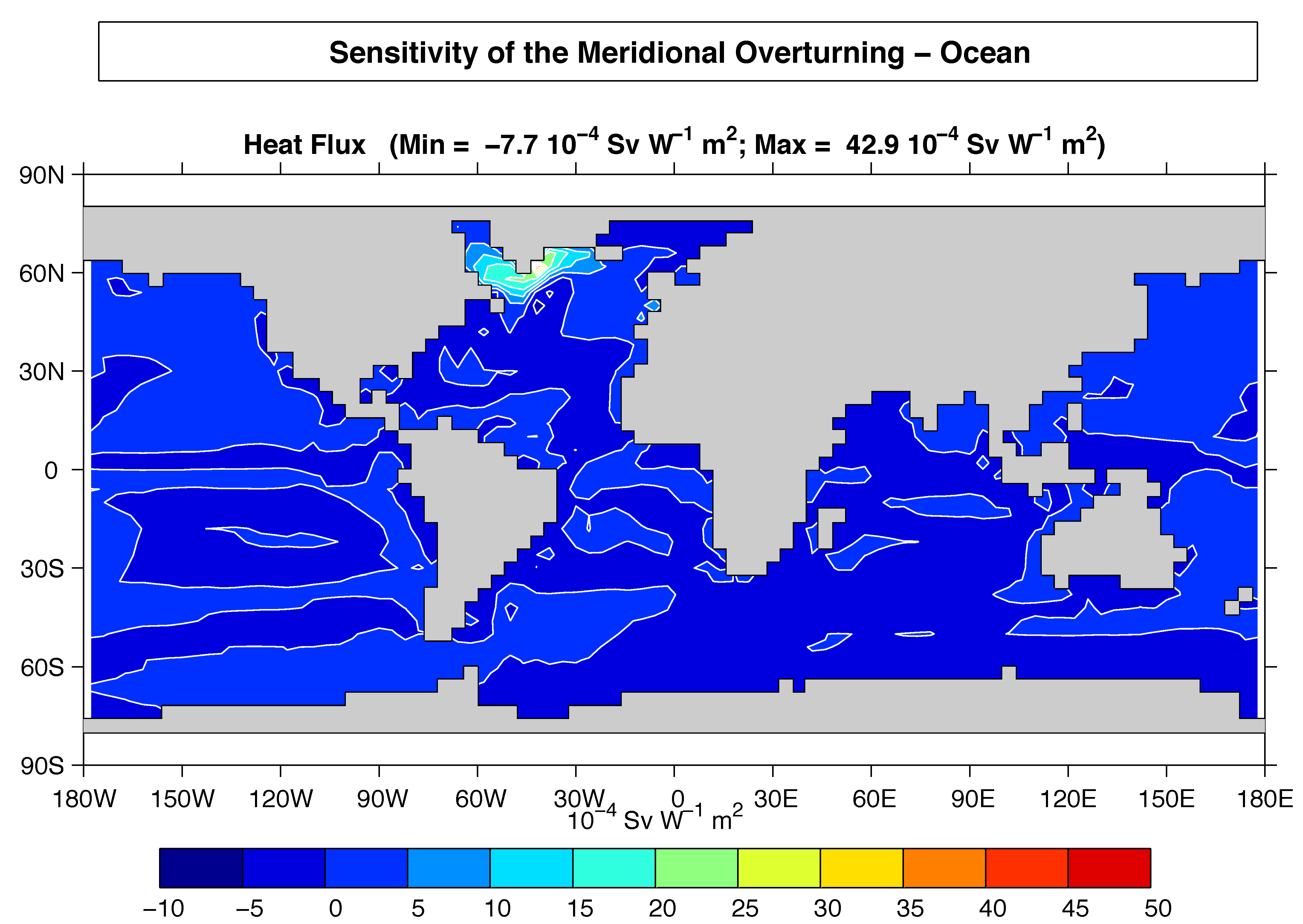# 1.2.6. Parameter sensitivity using the adjoint of MITgcm¶

Forward and tangent linear counterparts of MITgcm are supported using an ‘automatic adjoint compiler’. These can be used in parameter sensitivity and data assimilation studies.

As one example of application of the MITgcm adjoint, Figure 1.11 maps the gradient $$\frac{\partial J}{\partial\mathcal{H}}$$ where $$J$$ is the magnitude of the overturning stream-function shown in Figure 1.8 at 60°N and $$\mathcal{H}(\lambda,\varphi)$$ is the mean, local air-sea heat flux over a 100 year period. We see that $$J$$ is sensitive to heat fluxes over the Labrador Sea, one of the important sources of deep water for the thermohaline circulations. This calculation also yields sensitivities to all other model parameters.Figure 1.11 Sensitivity of meridional overturning strength to surface heat flux changes. Contours show the magnitude of the response (in Sv x 10-4 ) that a persistent +1 Wm-2 heat flux anomaly at a given grid point would produce.¶